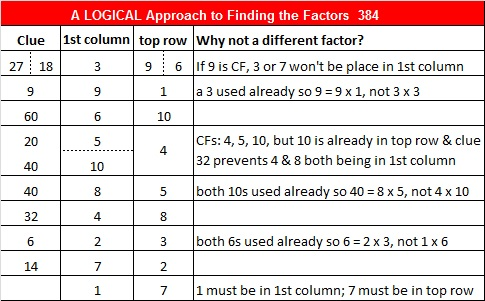# 384 and Level 6

384 is even and composite. Its factoring information is below the puzzle.Print the puzzles or type the factors on this excel file: 10 Factors 2015-02-02

• 384 is a composite number.
• Prime factorization: 384 = 2 x 2 x 2 x 2 x 2 x 2 x 2 x 3, which can be written 384 = (2^7) x 3
• The exponents in the prime factorization are 7 and 1. Adding one to each and multiplying we get (7 + 1)(1 + 1) = 8 x 2 = 16. Therefore 384 has exactly 16 factors.
• Factors of 384: 1, 2, 3, 4, 6, 8, 12, 16, 24, 32, 48, 64, 96, 128, 192, 384
• Factor pairs: 384 = 1 x 384, 2 x 192, 3 x 128, 4 x 96, 6 x 64, 8 x 48, 12 x 32, or 16 x 24
• Taking the factor pair with the largest square number factor, we get √384 = (√6)(√64) = 8√6 ≈ 18.596